### Home > CALC > Chapter 8 > Lesson 8.2.2 > Problem8-79

8-79.
1.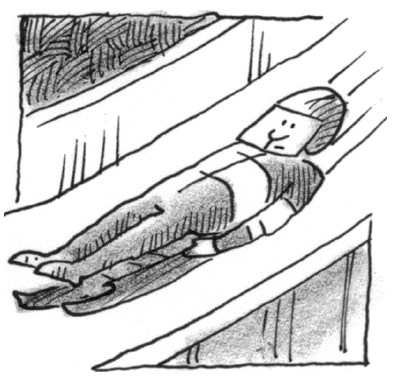One of the most popular events at the 1998 Winter Olympics in Nagano, Japan, was the luge. In this event, competitors lie on their backs and slide down an iced track on small sleds. At times, luge riders travel more than 120 km per hour!

2. During one particular run, a competitor from Norway had the times and distances listed in the table below. The track is 1200 m long. Enter the data points into your graphing calculator and then answer the following questions: Homework Help ✎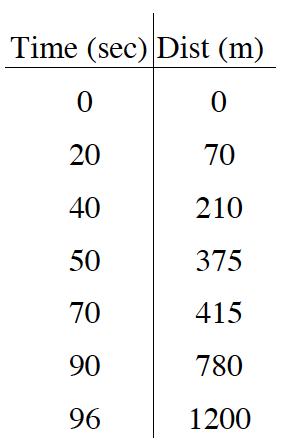1. Find the average velocity during the run.

2. The graph of the distance can be modeled by y = 0.000145x4 − 0.0246x3 + 1.315x2 − 15.808x + 5.582 using a quartic regression. Use your curve to approximate the velocity at t = 96 sec, when the athlete completed the race. Convert your result to km/hr. Is your result reasonable?

3. Graph the first derivative of your curve of best fit to represent the velocity. Explain its shape. What happens during the course of the run that helps explain its shape?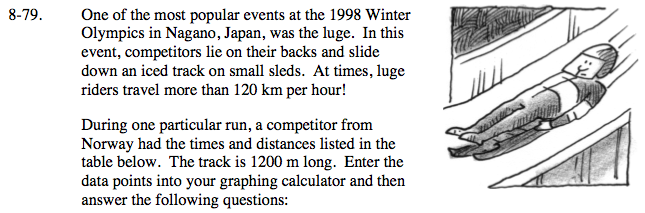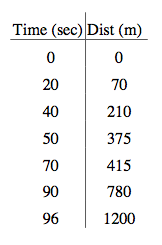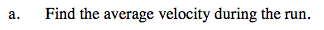Average velocity given position = AROC = slope of the secant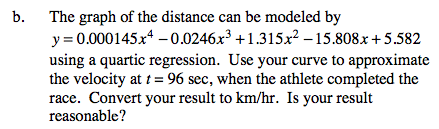If y = distance, what does y' = ?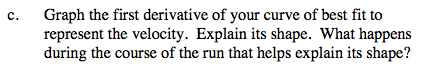Does the Norwegian competitor ride his luge at a constant rate? How can you tell?